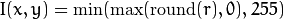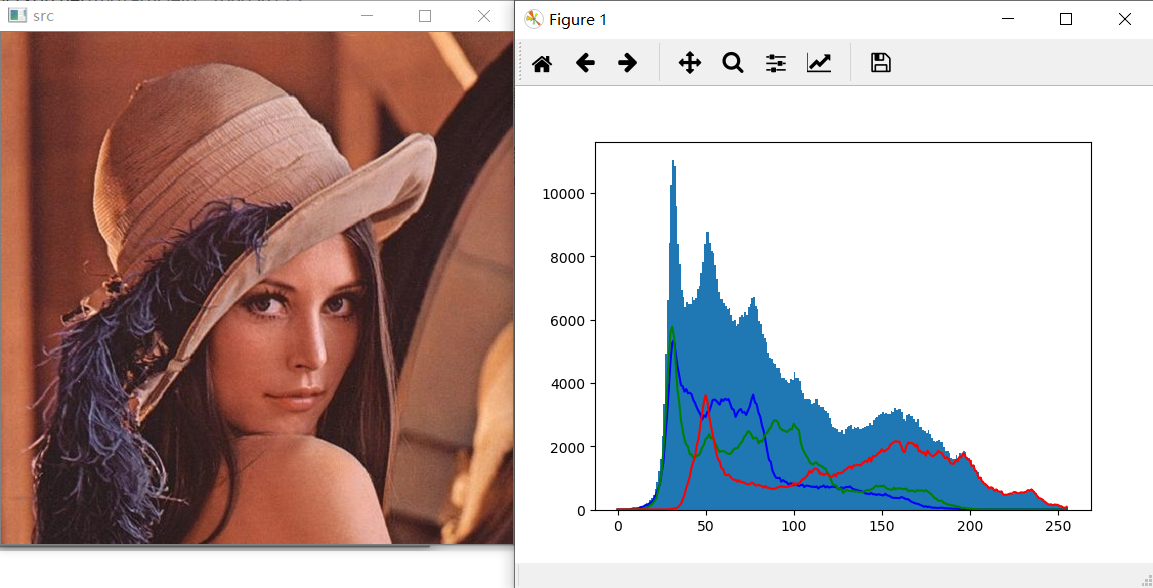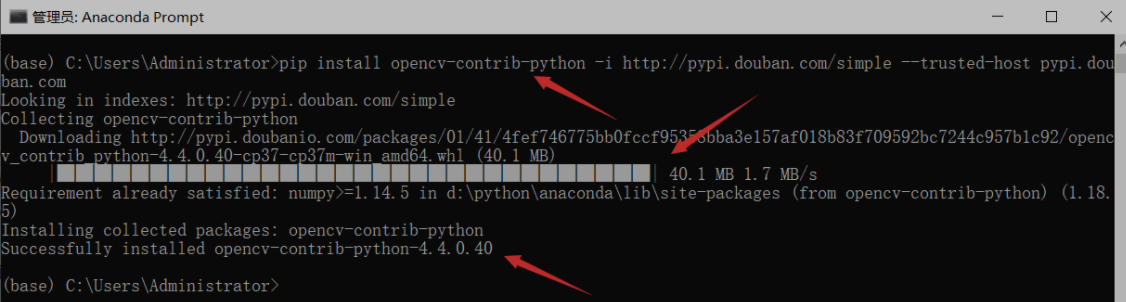# OpenCV基础介绍

+关注继续查看

Opencv 英文文档地址 ：  docs.opencv.org

OpenCV (Open Source Computer Vision Library: http://opencv.org) 是一个使用 BSD 许可证的开源库，包含数百个计算机视觉算法。此文档详细的描述了 OpenCV 2.x API，这主要是 C++ API，相对于 OpenCV 1.x API 的 C API。

OpenCV 使用模块化的结构，这表明其包含很多共享或者静态库。OpenCV 提供如下模块：

• core - 这是一个定义了基本数据结构的紧凑模块，包含大量被其他模块调用的多维的 Mat 和其他基本函数。
• imgproc - 这是一个图像处理模块，包含线性和非线性的图像过滤，几何图形转换 (大小调整, 仿射和透视扭曲, 通用的基于表的重新映射), 色彩空间转换以及直方图等等。
• video - 这是一个视频分析模块，包含运动评估、背景提取以及对象跟踪算法。
• calib3d - 基本的多视图几何算法，实现单个和立体的摄像头校准，对象构成的评估、立体相关算法和一些 3D 构造算法。
• features2d - 突出特征检测、描述以及描述器的匹配。
• objdetect - 对象检测以及预定义类的检测，例如人脸、眼睛、杯子、人物、汽车等等。
• highgui - 一个易用的视频捕获、图像和视频编码接口，同时提供简单的图形化界面。
• gpu - 来自不同 OpenCV 模块的 GPU 加速算法。
• ... 其他一些助手模块，例如 FLANN 和 Google 测试封装、Python 包等等。

## API 概念

### cv 命名空间

#include "opencv2/core/core.hpp"
...
cv::Mat H = cv::findHomography(points1, points2, CV_RANSAC, 5);
...


#include "opencv2/core/core.hpp"
using namespace cv;
...
Mat H = findHomography(points1, points2, CV_RANSAC, 5 );
...

Mat a(100, 100, CV_32F);
randu(a, Scalar::all(1), Scalar::all(std::rand()));
cv::log(a, a);
a /= std::log(2.);

### 自动内存管理

OpenCV 的内存处理是完全自动化的。

// 创建一个大的 8Mb 的矩阵
Mat A(1000, 1000, CV_64F);

// 创建该矩阵的另外一个引用
// 真实一个实例操作，无视阵列大小
Mat B = A;
// 为 A 的第三行创建另外一个头，此处没有数据拷贝动作
Mat C = B.row(3);
// 现在创建一个独立的阵列拷贝
Mat D = B.clone();
// 从 B 拷贝第五行数据到 C
// to the 3-rd row of A.
B.row(5).copyTo(C);
// 让 A 和 D 共享修改后数据，注意 A 仍被 B 和 C 引用
A = D;
// 现在让 B 变成空的阵列，释放内存,
// 但是修改好的 A 仍被 C 引用,
// 尽管 C 只是原始 A 的单行数据
B.release();

// 最，我们对 C 做一个全拷贝，这是大的改动
// 阵列将被释放，因为没有任何对象引用到它
C = C.clone();


T* ptr = new T(...);


Ptr<T> ptr = new T(...);


### 输出数据的自动分配

OpenCV 会自动释放内存，就如同大多数时候为输出函数的参数自动分配内存一样。因此，如果一个函数有一个或者多个输入的数组 (cv::Mat 实例) 和一些输出数组，输出的数组会实现自动的内存分配和释放。输出数组的大小和类型会根据输入数组的大小和类型来自动识别。如果需要的话函数可以提供额外的参数来帮助设定输出数组的属性。

#include "cv.h"
#include "highgui.h"

using namespace cv;

int main(int, char**)
{
VideoCapture cap(0);
if(!cap.isOpened()) return -1;

Mat frame, edges;
namedWindow("edges",1);
for(;;)
{
cap >> frame;
cvtColor(frame, edges, CV_BGR2GRAY);
GaussianBlur(edges, edges, Size(7,7), 1.5, 1.5);
Canny(edges, edges, 0, 30, 3);
imshow("edges", edges);
if(waitKey(30) >= 0) break;
}
return 0;
}


### 饱和算法I.at<uchar>(y, x) = saturate_cast<uchar>(r);


### 固定像素类型，模板使用限制

• 8-bit 无符号整数 (uchar)
• 8-bit 有符号整数 (schar)
• 16-bit 无符号整数 (ushort)
• 16-bit 有符号整数 (short)
• 32-bit 有符号整数 (int)
• 32-bit 浮点数(float)
• 64-bit 浮点数 (double)
• 一组多元素的元组，但所有元素的类型必须一致，而且必须是上面几种类型。数组的元素如果是元组，相当于是多通道数组，与单通道数组也跟元组类型相反，这些元素必须是标量类型。最大的通道数是定义为 CV_CN_MAX 的常量值，当前是 512.

enum { CV_8U=0, CV_8S=1, CV_16U=2, CV_16S=3, CV_32S=4, CV_32F=5, CV_64F=6 };


• CV_8UC1 ... CV_64FC4 常量 (对应 1 到 4 的通道编号)
• CV_8UC(n) ... CV_64FC(n) 或者 CV_MAKETYPE(CV_8U, n) ... CV_MAKETYPE(CV_64F, n) 宏，当通道数量超过 4 或者未知时

CV_32FC1 == CV_32F, CV_32FC2 == CV_32FC(2) == CV_MAKETYPE(CV_32F, 2), 以及 CV_MAKETYPE(depth, n) == (depth&7) + ((n-1)<<3). 意思是常量类型是根据深度形成的。占用了低位 3 比特，此外通道数量减1占用下一个 log2(CV_CN_MAX) 比特.

Mat mtx(3, 3, CV_32F); // 生成一个 3x3 浮点阵列
Mat cmtx(10, 1, CV_64FC2); // 生成一个 10x1 2通道浮点
// 阵列 (10元素的复杂向量)
Mat img(Size(1920, 1080), CV_8UC3); // 生成一个三通道的彩色图像
// 包含 1920 列和 1080 行.
Mat grayscale(image.size(), CV_MAKETYPE(image.depth(), 1)); // 生成一个单通道的相同大小和通道类型的图像


• 人脸识别算法只支持 8 位灰度图以及彩色图.
• 线性代数函数以及大多数的机器学习算法只支持浮点数数组.
• 色彩空间转换函数支持 8位无符号、16位无符号以及32位浮点数类型.

### 错误处理

OpenCV 使用异常来表示关键错误。当输入的数据包含正确的格式以及属于指定的值范围，但是算法因为某种原因无法正确处理时就会返回特定的错误码（一般是一个布尔值变量）。

try
{
... // call OpenCV
}
catch( cv::Exception& e )
{
const char* err_msg = e.what();
std::cout << "exception caught: " << err_msg << std::endl;
}


### 多线程和可重入|

opencv图像处理基础(python)
opencv图像处理基础(python)
132 0
|

Python opencv图像处理基础总结(七) 基于分水岭算法的图像分割

249 0|

Python opencv图像处理基础总结(三) 图像直方图 直方图应用 直方图反向投影

373 0|

Python opencv图像处理基础总结(一) 环境搭建 基础操作
Open Source Computer Vision Library，OpenCV于 1999 年由 Intel 建立，如今由 Willow Garage 提供支持。OpenCV是一个基于 BSD 许可(开源)发行的跨平台计算机视觉库，可以运行在Linux、Windows、MacOS操作系统上。
176 0|
Python 计算机视觉
Python+OpenCV教程基础篇：图像混合

1348 0
|

OpenCV 估算图像的投影关系：基础矩阵和RANSAC

1992 0